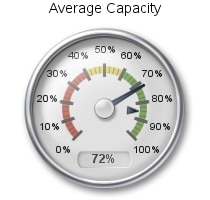# Example 4: Defining a Speedometer

 Features: PROC GKPI statement option: MODE=RAISED SPEEDOMETER statement options: COLORS= FORMAT= LABEL= LFONT= TARGET= Sample library member: GKPSPD## Program

`goptions reset=all device=javaimg xpixels=210 ypixels=200;`
```proc gkpi mode=raised;
speedometer actual=.72 bounds=(0 .40 .60 1) /   target=.85
lfont=(f="Albany AMT" height=.5cm) label="Average Capacity"
format="percent8.0";
run;```
`quit;`

## Program Description

Set the graphics environment.The XPIXELS and YPIXELS graphics options reduce the size of the graphics output area and, therefore, reduce both the size of the KPI chart and the distance between the label and the KPI chart. These options scale the KPI charts to a size that would be appropriate for use in a dashboard.
`goptions reset=all device=javaimg xpixels=210 ypixels=200;`
Generate the KPI chart. Specify the range boundaries, actual KPI value, and target value. The LFONT= option specifies the font for the label. The FORMAT= option specifies the SAS format for the values in the chart.
```proc gkpi mode=raised;
speedometer actual=.72 bounds=(0 .40 .60 1) /   target=.85
lfont=(f="Albany AMT" height=.5cm) label="Average Capacity"
format="percent8.0";
run;```
End the procedure. The GKPI procedure supports RUN-group processing, so it is recommended that you enter the QUIT statement to end the procedure.
`quit;`